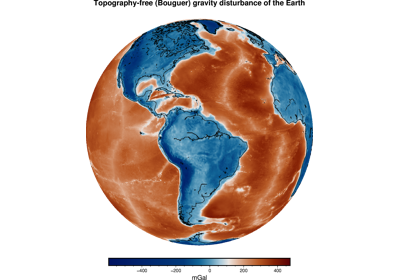# harmonica.bouguer_correction¶

harmonica.bouguer_correction(topography, density_crust=2670, density_water=1040)[source]

Gravitational effect of topography using a Bouguer plate approximation

Used to remove the gravitational attraction of topography above the ellipsoid from the gravity disturbance. The infinite plate approximation is adequate for regions with flat topography and observation points close to the surface of the Earth.

This function calculates the classic Bouguer correction:

$g_{bg} = 2 \pi G \rho h$

in which $$G$$ is the gravitational constant and $$g_{bg}$$ is the gravitational effect of an infinite plate of thickness $$h$$ and density $$\rho$$.

In the oceans, subtracting normal gravity from the observed gravity results in over correction because the normal Earth has crust where there was water in the real Earth. The Bouguer correction for the oceans aims to remove this residual effect due to the over correction:

$g_{bg} = 2 \pi G (\rho_w - \rho_c) |h|$

in which $$\rho_w$$ is the density of water and $$\rho_c$$ is the density of the crust of the normal Earth. We need to take the absolute value of the bathymetry $$h$$ because it is negative and the equation requires a thickness value (positive).

Parameters
• topography (array or xarray.DataArray) – Topography height and bathymetry depth in meters. Should be referenced to the ellipsoid (ie, geometric heights).

• density_crust (float) – Density of the crust in $$kg/m^3$$. Used as the density of topography on land and the density of the normal Earth’s crust in the oceans.

• density_water (float) – Density of water in $$kg/m^3$$.

Returns

grav_bouguer (array or xarray.DataArray) – The gravitational effect of topography and residual bathymetry in mGal.

## Examples using harmonica.bouguer_correction¶